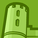# QlikView App Dev

Discussion Board for collaboration related to QlikView App Development.

Announcements
Skip the ticket, Chat with Qlik Support instead for instant assistance.
cancel
Showing results for
Did you mean:Contributor

## Scatter Chart – mouse over popup lines restrictions.

I’ve Scatter Chart with 2 dimensions (i.e. Member ID, Member Name) and 5 expressions (i.e. Category-1 to Category-5) and when I mouse over on chart bubble 2+5 i.e. 7 lines are displaying on popup menu as below

Member ID = 100

Member Name = David

Category-1 = A

Category-2 = B

Category-3 = C

Category-4 = D

Category-5 = E

For the count purpose I’ve added the Category-4 and Category-5 expressions as a mandatory, however I would like to exclude those two of the expression lines on mouse over popup, means I would like to see only first 5 lines instead of 7 lines on mouse over popup as below –

Member ID = 100

Member Name = David

Category-1 = A

Category-2 = B

Category-3 = C

The Text as Pop-up will work but only, I can see the values, where as I would like to see the value along with labels as well.

Will you please help, how to customize the Scatter Chart mouse over lines to exclude to display the below  -

Category-4 = D

Category-5 = E

2 Solutions

Accepted SolutionsMVP

Hi, you can create the expression with the text included to make it look like the others, so instead of =Sum(Value) the expression can be: ='Category 4 = ' & Sum(Value).

You can also use Dual() to set the text to show and the value to apply to the chart, ie.: Dual('Category 4 = ' & Sum(Value), Sum (Value))MVP

I have no direct solution for this one, an alternative can be having a table near the scatter chart that allows for exporting in table format.

6 RepliesMVP

Hi, you can create the expression with the text included to make it look like the others, so instead of =Sum(Value) the expression can be: ='Category 4 = ' & Sum(Value).

You can also use Dual() to set the text to show and the value to apply to the chart, ie.: Dual('Category 4 = ' & Sum(Value), Sum (Value))Contributor
Author

Thanks Rubenmarin,

Yeah it is working as expected for the expression lines to restrict, in this way I'm loosing the dimension labels.

With this scenario, how to display the dimension labels along with expression label restrictions.   I would like to display the below 2 dimension and 3 expression labels on popup total 5 lines instead of 7 lines (restriction of 2 expression labels).

Member ID = 100      -  (dimension label)

Member Name = David  -  (dimension label)

Category-1 = A   -  (expression label)

Category-2 = B   -  (expression label)

Category-3 = C   -  (expression label)MVP

Maybe using Dual() in first expression to show dimension values, ie:

Dual('Dimension1 = ' & dimensionfield1 & Chr(10) & 'Dimension2 = ' & dimensionfield2 & Chr(10) & 'Expression1 = ' & Sum(Value), Sum (value))Contributor
Author

Thanks a lot Rubenmarin, as you suggested I've made the dimensions into first expression and it is working perfect what I was looking.

Appreciated on your very quick support.Contributor
Author

The solution is working perfect at dashboard level, when I send to excel from chart values, the Member ID column value displaying both dimension and first expression values as below –

Member ID = 100 Member Name = David Category-1 = A

Also the Category-2 Column value as Category-2 = B and the similar for Category-3.

Is there any alternate solution, when send to excel to display the proper column values to avoid the concatenated values?  i.e. instead of above display in excel column values as below -

Member ID = 100

Member Name = David

Category-1 = A

Category-2 = B

Category-3 = CMVP

I have no direct solution for this one, an alternative can be having a table near the scatter chart that allows for exporting in table format.Community Browser